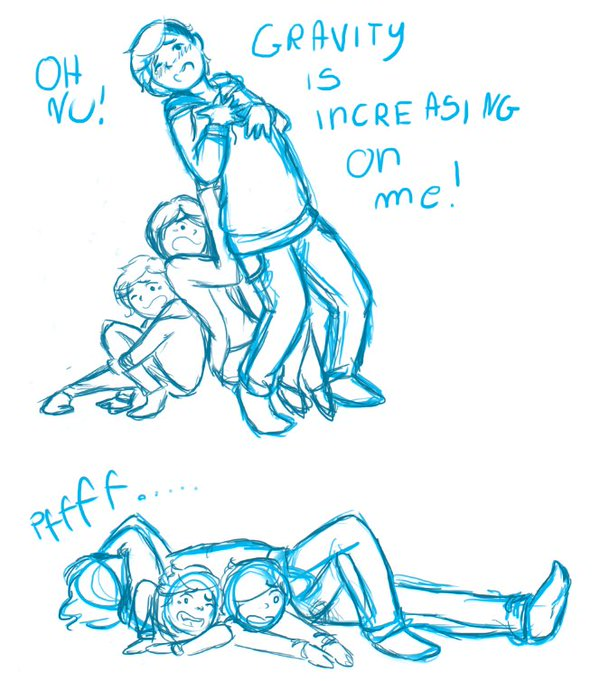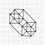# What if gravity was $9.8 m s^{-3}$?

I was thinking about this for a while. Clearly, if gravity was just 9.8m/s (velocity), we would all feel a sudden tug and then infinite weightlessness. But what if gravity turned into jerk?

Would it be possible to fly?

Theoretically, could you jump higher?

How much energy would it take to escape earth's orbit? Could you even escape?

How long until the earth crashed into the sun?

Of course, since gravity would constantly increase, if all other forces stayed the same, we would probably just slowly be crushed ): So assume gravity starts at 0m/s^2 when answering the above problems.

But here's another question. What if ALL forces were in terms of jerk instead of acceleration? What would that look like? Would it simply seem exactly the same as before?

What about if everything went up a derivative?

What if everything except light-speed went up a derivative?Note by Alex Li
4 years, 7 months ago

This discussion board is a place to discuss our Daily Challenges and the math and science related to those challenges. Explanations are more than just a solution — they should explain the steps and thinking strategies that you used to obtain the solution. Comments should further the discussion of math and science.

When posting on Brilliant:

• Use the emojis to react to an explanation, whether you're congratulating a job well done , or just really confused .
• Ask specific questions about the challenge or the steps in somebody's explanation. Well-posed questions can add a lot to the discussion, but posting "I don't understand!" doesn't help anyone.
• Try to contribute something new to the discussion, whether it is an extension, generalization or other idea related to the challenge.

MarkdownAppears as
*italics* or _italics_ italics
**bold** or __bold__ bold
- bulleted- list
• bulleted
• list
1. numbered2. list
1. numbered
2. list
Note: you must add a full line of space before and after lists for them to show up correctly
paragraph 1paragraph 2

paragraph 1

paragraph 2

[example link](https://brilliant.org)example link
> This is a quote
This is a quote
    # I indented these lines
# 4 spaces, and now they show
# up as a code block.

print "hello world"
# I indented these lines
# 4 spaces, and now they show
# up as a code block.

print "hello world"
MathAppears as
Remember to wrap math in $$ ... $$ or $ ... $ to ensure proper formatting.
2 \times 3 $2 \times 3$
2^{34} $2^{34}$
a_{i-1} $a_{i-1}$
\frac{2}{3} $\frac{2}{3}$
\sqrt{2} $\sqrt{2}$
\sum_{i=1}^3 $\sum_{i=1}^3$
\sin \theta $\sin \theta$
\boxed{123} $\boxed{123}$

Sort by:

Did you know that constant acceleration is indistinguishable from being in a gravitational field? If that were not true, then General Relativity would not hold.

- 4 years, 7 months ago

No, but you ruined it :( I'm not asking if it's possible (clearly it's not), I'm asking what it would be like if it were true.

- 4 years, 7 months ago

Everyone dies! Because, at this rate the jerk would cause an enormous amount of acceleration leading the Earth to become a black hole.

- 4 years, 7 months ago

If you mean the acceleration of the earth is increasing, with some constant jerk, then first everything on the earth would concentrate itself into a point mass at the center of mass of the earth. Now if your saying all forces would go up a derivative, then first the solar system would converge at its center of mass, then the solar system would converge into some other center of mass of some other system which in turns converges into some other center of mass and this would go on till once again the big bang would be formed from which all started. Maybe, its just a thought. Everything in the universe would converge into the center of mass because it would attain maximu stability and thus least potential energy.

- 4 years, 7 months ago